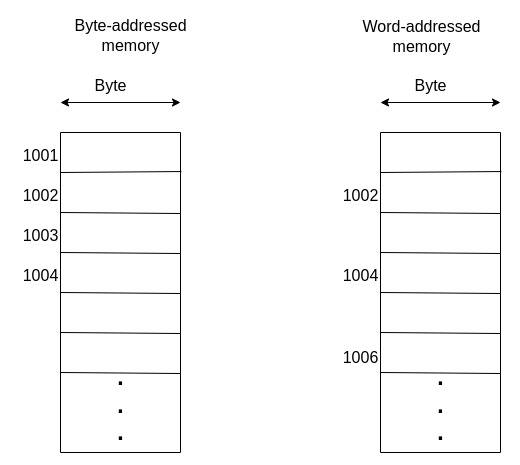## 1. Introduction

Memory addresses are references used to identify the location of data or instructions when a program is running. They are virtual memory locations used to map logical addresses to the physical ones on the computer.

Logical addresses are used to specify the address space for a program. Address space refers to the range of virtual addresses allocated to a process.

The way in which memory is addressed has various implications on the memory access patterns and the way it’s stored.

In this tutorial, we’ll examine how byte-addressed memory differs from word-addressed memory.

A byte (8 bits) is the minimum addressable unit on modern-day computers. The number of addresses a processor can access is determined by its bit size. A 32-bit processor can, for example, accessaddresses(about 4GB). For a 64-bit processor, the value is.

A word is a group of bytes. Therefore, a word can be 16 bits, 24 bits, 32 bits, and so on.

Words are normally used to store instructions for the CPU, most commonly 32 bits. Each segment of the 32-bit instruction represents some important information.

However, sometimes words can be used to represent data. Data types like integer and double-precision floating-point are normally 16-bit and 64-bit words, respectively.

When specifying an address for words, we need to look at the number of bytes they contain. We’ll explore this further below.

## 4. Endianness

Endianness refers to the order in which bytes are arranged in memory. If the bytes are arranged from the most significant to least significant byte, we call that byte order big-endian. This is the arrangement that we naturally use.

Conversely, if the bytes are arranged from the least significant to the most significant, we call it little-endian.

Normally, we don’t have to worry about what type of ordering our CPU uses. However, knowing the byte ordering used might be useful if we’re building a network application. For example, Intel processors normally use little-endian while IBM processors use big-endian. Depending on the application, this can affect how data is transferred over a network.

TCP/IP networks typically use big-endian. The byte sequence used is commonly referred to as network byte order. For Web3, it’s important to note that blockchains like Bitcoin and Ethereum use little-endian network byte order.

Depending on the program, the type of endianness can also bring substantial memory savings and performance benefits. Little-endian byte ordering generally saves you some data since it begins with the least significant bit. We don’t need to rearrange the byte order when the value increases. We only add the new value to the left.

Note that the bit order within each byte remains big-endian in little-end and big-endian sequences.

## 5. Alignment

When dealing with byte-addressed memory, each byte represents an independent datum. The same isn’t true for word-addressed memory. Instead, a collection of bytes does. This, therefore, affects how we access the data.

First, it’s important to understand that processes view addresses as consecutive blocks of bytes.

Let’s say that we are storing floating point values using 16-bit values. The memory access pattern for this data is supposed to be 0, 2, 4, and so on.

The data would be misaligned if we accessed memory locations from values such as 3, 5, and 7. That is, we would get improper data, as seen below:For this reason, it is necessary to consider alignment issues while accessing word-addressed memory. A helpful formula we can use to ensure that we are accessing the right addresses is:, whereis the byte address andis the word size.

## 4. Conclusion

In this tutorial, we have learned how the addressing effects can affect various aspects, such as byte ordering and memory access patterns.

Subscribe
Notify of